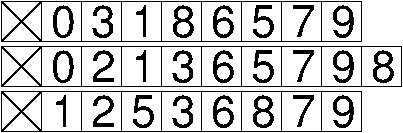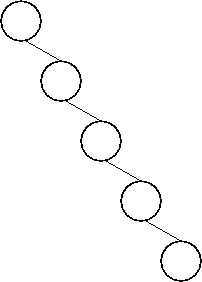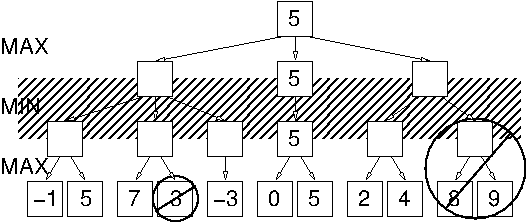CS 61B: Data Structures (Spring 2014)
Midterm II

### Solutions

Problem 1. (10 points) Trees.

a. Many students provided answers in which some of the leaves are at a greater depth than other leaves. If you learn only one thing about 2-3-4 trees, know that all the leaves must be at the same depth!b. The smallest possible number of keys in a valid 2-3-4 tree of depth 3 is 15.

c.d. The total running time for all n insertion operations together is in Θ(n), because each newly inserted key stays at the bottom level of the tree and does not bubble up (being the largest key in the tree), so each insertion operation takes constant time.

e. The total running time for all n removal operations together is in Θ(n log n).

f. The total running time for all n insertion operations together is in Θ(n2). The tree looks like this.g. The total running time for all n removal operations together is in Θ(n), because each remove() operation removes a root that has only one child, which is done in constant time.

Problem 2. (7 points) Asymptotic Analysis.

a. O(9x + log z + y √log z + y2 / z2).

b. (i) False. A counterexample is f(n) = 2n; g(n) = n. Clearly f(n) ∈ O(g(n)), but 2f(n) = 4n ∉ O(2n) = O(2g(n)), because 4n is larger than 2n by a factor of 2n (which is not a constant).

b. (ii) True. We assume that f(n) ∈ O(g(n)), which means that there exist constants c and N such that for all nN, f(n) ≤ c g(n).

It follows that for all nN, log f(n) ≤ log c + log g(n). Let N' be a number such that for all nN', g(n) ≥ c. Then for all n ≥ max{N, N'}, log f(n) ≤ 2 log g(n), which proves that log f(n) ∈ O(log g(n)).

Problem 3. (8 points) Algorithms.

a. The score of the root node is 5.b. The fastest possible algorithm to answer this question takes Θ(k2) time with an adjacency list (using linked lists), and Θ(n) time with an adjacency matrix.

c. Note: many students didn't read this question carefully enough. The graph does not come with color preassigned to the vertices; it's your job to pick a color for each vertex.

Pick an arbitrary starting vertex and assign it an arbitrary color (red or blue). Perform a depth-first search or a breadth-first search from that vertex. Whenever you visit a new vertex for the first time, assign it the color opposite of the color of the last vertex you just came from. Whenever you follow an edge and find a vertex that has already been visited, check its color; if it has the same color as the last vertex you just came from, output a message saying that the graph is not bipartite and halt.

If the DFS or BFS finishes without halting early, the graph is bipartite.

d. Before answering the question, I want to comment on what makes this question hard. Many students claimed that it is necessary to check every edge of the graph, but that's not true. If the graph is not bipartite, you can stop checking edges as soon as you identify one cycle with an odd number of edges. Whether the graph is bipartite or not, here's a clever trick that allows you to skip looking at a few of the edges. Do a DFS, but stop it short as soon as you visit the last vertex, which we'll call v. At this point, you have assigned colors to all the vertices, but you've inspected only one of the edges adjoining v. You can avoid inspecting any other edge adjoining v as follows. Suppose that v is red. Then inspect all the edges out of all the red vertices except v. (Note: no need to re-inspect outbound edges already inspected by DFS.) If all those edges lead to blue vertices, then you know that v doesn't adjoin a red vertex either, even without inspecting any edge adjoining v (other than the edge through which we found v). If the total degree of all the blue vertices equals the total degree of all the red vertices, then you also know that no blue vertex adjoins a blue vertex, so the graph is bipartite. (Observe that the vertex degrees give us information that permits us to avoid looking at some of the edges.)

Now let's answer the exam question. If it isn't necessary to inspect every edge, which edges must you inspect?

Consider a graph G that is bipartite, and suppose that it is “sufficiently well-connected” that you cannot disconnect it by removing two edges. Consider an algorithm (any correct algorithm whatsoever) that determines whether G is bipartite. Suppose there are two edges that the algorithm has not looked at, (r1, b1) and (r2, b2), where r1 and r2 are two different red vertices, and b1 and b2 are two different blue vertices. If you remove those two edges and replace them with (r1, r2) and (b1, b2), you obtain a modified graph G' that is not bipartite. (Note that we cannot change the coloring of the vertices, because we assumed that removing two edges from G does not disconnect it.) However, observe that there is no other difference between the data structures representing G and G' besides four changed destination vertices in the adjacency list: two changed edges times two directions. In particular, no vertex degree has changed, and the adjacency list records the same edges in the same order except that four destination vertices have changed. Therefore, we absolutely cannot distinguish G from G' without inspecting one of the two edges. Every correct algorithm must inspect at least one of them.

To be certain that a “sufficiently well-connected” graph is bipartite, we must keep inspecting edges until there are no two uninspected edges that don't share a common vertex. We can stop inspecting edges only when every edge still uninspected adjoins a common vertex v. Therefore, we must inspect at least en + 1 edges (because the degree of v is at most n). If e ≥ 2n, this is Ω(e) edges, so the algorithm takes Ω(e) time. If e < 2n, it takes Ω(n) time just to inspect each vertex, which is at least Ω(e) time.

Now that you've seen a rigorous answer, you might wonder what answers actually received credit on the exam. (Obviously, they weren't this long.) We didn't give credit for claiming that it is necessary to inspect every edge of the graph—partly because that's wrong, but also because we only give credit for attempting to prove that we must inspect most of the edges. We looked for answers that point out that you can transform a bipartite graph to a non-bipartite graph by changing just an edge or two, and that there are many such transformations that can be made to the same bipartite graph.

Mail inquiries to cs61b@cory.eecs